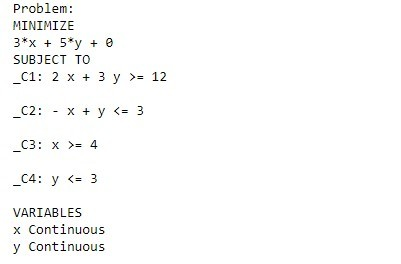# Python | Linear Programming in Pulp

Linear Programming (LP), also known as linear optimization is a mathematical programming technique to obtain the best result or outcome, like maximum profit or least cost, in a mathematical model whose requirements are represented by linear relationships. Linear programming is a special case of mathematical programming, also known as mathematical optimization.
Generally, an organization or a company has mainly two objectives, the first one is minimization and the other is maximization. Minimization means to minimize the total cost of production while maximization means to maximize their profit. So with the help of linear programming graphical method, we can find the optimum solution.

Basic terminologies of Linear Programming

• Objective Function: The main aim of the problem, either to maximize of to minimize, is the objective function of linear programming. In the problem shown below, Z (to minimize) is the objective function.
• Decision Variables: The variables used to decide the output as decision variables. They are the unknowns of the mathematical programming model. In the below problem, we are to determine the value of x and y in order to minimize Z. Here, x and y are the decision variables.
• Constraints: These are the restrictions on the decision variables. The limitations on the decision variables given under subject to the constraints in the below problem are the constraints of the Linear programming.
• Non – negativity restrictions: In linear programming, the values for decision variables are always greater than or equal to 0.

Note: For a problem to be a linear programming problem, the objective function, constraints, and the non – negativity restrictions must be linear.

Example 1: Consider the following problem:

```Minimize :  Z = 3x + 5y
Subject to the constraints:
2x + 3y >= 12
-x + y <= 3
x >= 4
y <= 3
x, y >= 0
```

Solving the above linear programming problem in Python:
PuLP is one of many libraries in Python ecosystem for solving optimization problems. You can install PuLp in Jupyter notebook as follows:

 `import` `sys !{sys.executable} ``-``m pip install pulp `

Code : To solve the aforementioned linear programming problem in Python:

 `# import the library pulp as p ` `import` `pulp as p ` ` `  `# Create a LP Minimization problem ` `Lp_prob ``=` `p.LpProblem(``'Problem'``, p.LpMinimize)  ` ` `  `# Create problem Variables  ` `x ``=` `p.LpVariable(``"x"``, lowBound ``=` `0``)   ``# Create a variable x >= 0 ` `y ``=` `p.LpVariable(``"y"``, lowBound ``=` `0``)   ``# Create a variable y >= 0 ` ` `  `# Objective Function ` `Lp_prob ``+``=` `3` `*` `x ``+` `5` `*` `y    ` ` `  `# Constraints: ` `Lp_prob ``+``=` `2` `*` `x ``+` `3` `*` `y >``=` `12` `Lp_prob ``+``=` `-``x ``+` `y <``=` `3` `Lp_prob ``+``=` `x >``=` `4` `Lp_prob ``+``=` `y <``=` `3` ` `  `# Display the problem ` `print``(Lp_prob) ` ` `  `status ``=` `Lp_prob.solve()   ``# Solver ` `print``(p.LpStatus[status])   ``# The solution status ` ` `  `# Printing the final solution ` `print``(p.value(x), p.value(y), p.value(Lp_prob.objective))   `

### Explanation :

Now, let’s understand the code step by step:

• Line 1-2: First import the library pulp as p.
• Line 4-5: Define the problem by giving a suitable name to your problem, here I have given the name ‘Problem’. Also, specify your aim for the objective function of whether to Maximize or Minimize.
• Line 7-9: Define LpVariable to hold the variables of the objective functions. The next argument specifies the lower bound of the defined variable, i.e. 0, and the upper bound is none by default. You can specify the upper bound too.
• Line 11-12: Denotes the objective function in terms of defined variables.
• Line 14-18: These are the constraints on the variables.
• Line 21: This will show you the problem in the output screen.
• Line 23: This is the problem solver.
• Line 24: Will display the status of the problem.
• Line 27: Will print the value for x and y and the minimum value for the objective function.

Review the Output

 `# Display the problem ` `print``(Lp_prob) `

Output`status ``=` `Lp_prob.solve()   ``# Solver ` `print``(p.LpStatus[status])   ``# The solution status `

Output

`Optimal`

 `# Printing the final solution ` `print``(p.value(x), p.value(y), p.value(Lp_prob.objective)) `

Output

`6.0 0.0 18.0`

The optimal value for x and y are 6.0 and 0.0 respectively. The optimised objective function value is 18.0.

My Personal Notes arrow_drop_upPossess good Mathematical and Statistical Foundation Data Science Enthusiast Addicted to Python

If you like GeeksforGeeks and would like to contribute, you can also write an article using contribute.geeksforgeeks.org or mail your article to contribute@geeksforgeeks.org. See your article appearing on the GeeksforGeeks main page and help other Geeks.

Please Improve this article if you find anything incorrect by clicking on the "Improve Article" button below.

Article Tags :

2

Please write to us at contribute@geeksforgeeks.org to report any issue with the above content.